# Yii - Query Builder

Query builder allows you to create SQL queries in a programmatic way. Query builder helps you write more readable SQL-related code.

To use query builder, you should follow these steps −

• Build an yii\db\Query object.
• Execute a query method.

To build an yii\db\Query object, you should call different query builder functions to define different parts of an SQL query.

Step 1 − To show a typical usage of the query builder, modify the actionTestDb method this way.

```public function actionTestDb() {
//generates "SELECT id, name, email FROM user WHERE name = 'User10';"
\$user = (new \yii\db\Query())
->select(['id', 'name', 'email'])
->from('user')
->where(['name' => 'User10'])
->one();
var_dump(\$user);
}
```

Step 2 − Go to http://localhost:8080/index.php?r=site/test-db, you will see the following output.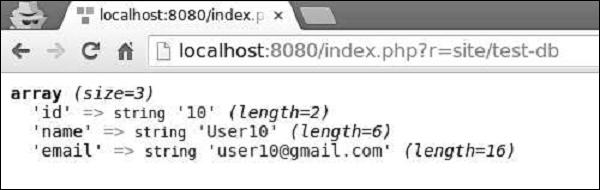## Where() function

The where() function defines the WHERE fragment of a query. To specify a WHERE condition, you can use three formats.

• string format − 'name = User10'

• hash format − ['name' => 'User10', 'email => user10@gmail.com']

• operator format − ['like', 'name', 'User']

### Example of String format

```public function actionTestDb() {
\$user = (new \yii\db\Query())
->select(['id', 'name', 'email'])
->from('user')
->where('name = :name', [':name' => 'User11'])
->one();
var_dump(\$user);
}
```

Following will be the output.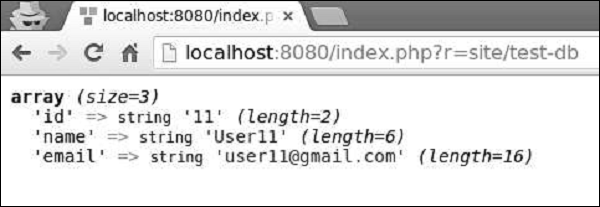### Example of Hash format

```public function actionTestDb() {
\$user = (new \yii\db\Query())
->select(['id', 'name', 'email'])
->from('user')
->where([
'name' => 'User5',
'email' => 'user5@gmail.com'
])
->one();
var_dump(\$user);
}
```

Following will be the output.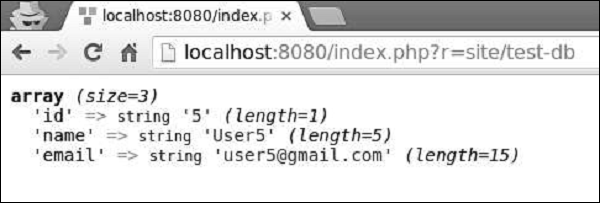Operator format allows you to define arbitrary conditions in the following format −

```[operator, operand1, operand2]
```

The operator can be −

• and − ['and', 'id = 1', 'id = 2'] will generate id = 1 AND id = 2 or: similar to the and operator

• between − ['between', 'id', 1, 15] will generate id BETWEEN 1 AND 15

• not between − similar to the between operator, but BETWEEN is replaced with NOT BETWEEN

• in − ['in', 'id', [5,10,15]] will generate id IN (5,10,15)

• not in − similar to the in operator, but IN is replaced with NOT IN

• like − ['like', 'name', 'user'] will generate name LIKE '%user%'

• or like − similar to the like operator, but OR is used to split the LIKE predicates

• not like − similar to the like operator, but LIKE is replaced with NOT LIKE

• or not like − similar to the not like operator, but OR is used to concatenate the NOT LIKE predicates

• exists − requires one operand which must be an instance of the yii\db\Query class

• not exists − similar to the exists operator, but builds a NOT EXISTS (subquery) expression

• <, <=, >, >=, or any other DB operator: ['<', 'id', 10] will generate id<10

### Example of Operator format

```public function actionTestDb() {
\$users = (new \yii\db\Query())
->select(['id', 'name', 'email'])
->from('user')
->where(['between', 'id', 5, 7])
->all();
var_dump(\$users);
}
```

Following will be the output.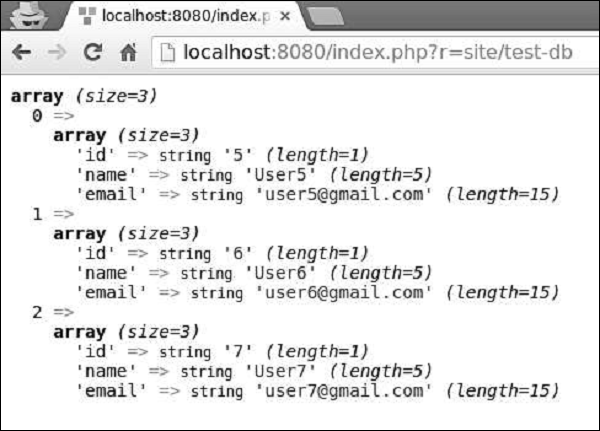## OrderBy() Function

The orderBy() function defines the ORDER BY fragment.

Example

```public function actionTestDb() {
\$users = (new \yii\db\Query())
->select(['id', 'name', 'email'])
->from('user')
->orderBy('name DESC')
->all();
var_dump(\$users);
}
```

Following will be the output.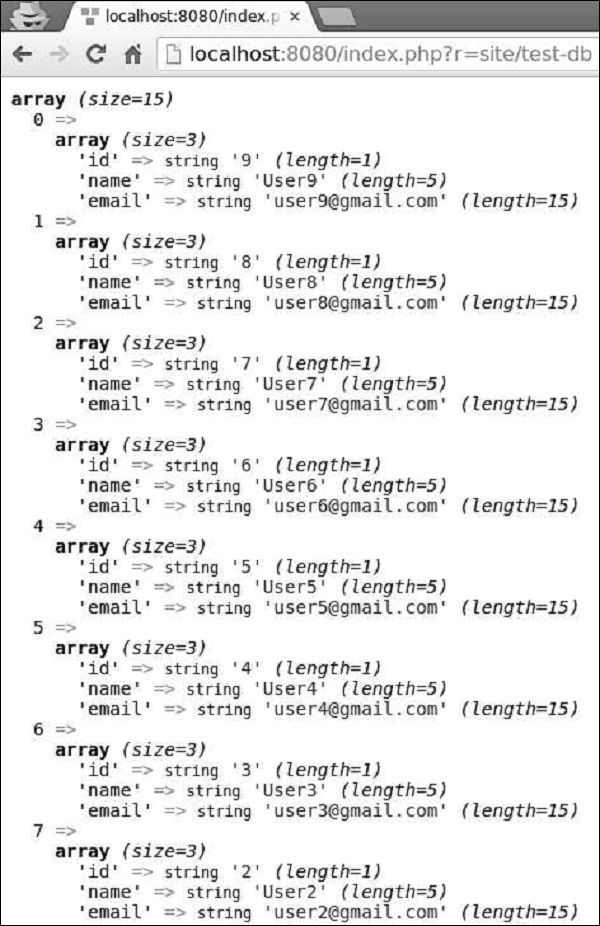## groupBy() Function

The groupBy() function defines the GROUP BY fragment, while the having() method specifies the HAVING fragment.

Example

```public function actionTestDb() {
\$users = (new \yii\db\Query())
->select(['id', 'name', 'email'])
->from('user')
->groupBy('name')
->having('id < 5')
->all();
var_dump(\$users);
}
```

Following will be the output.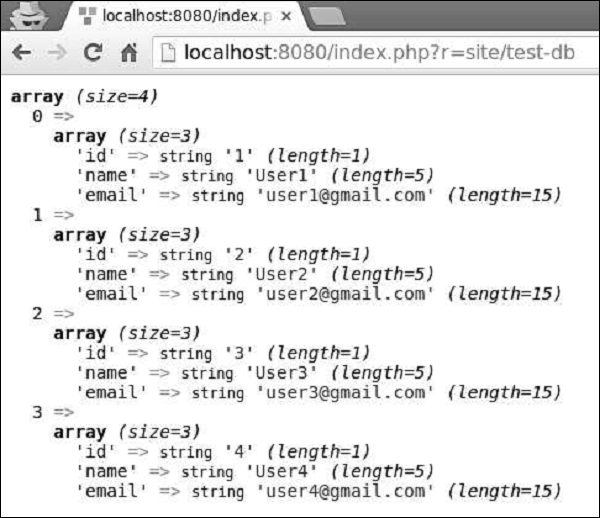The limit() and offset() methods defines the LIMIT and OFFSET fragments.

Example

```public function actionTestDb() {
\$users = (new \yii\db\Query())
->select(['id', 'name', 'email'])
->from('user')
->limit(5)
->offset(5)
->all();
var_dump(\$users);
}
```

You can see the following output −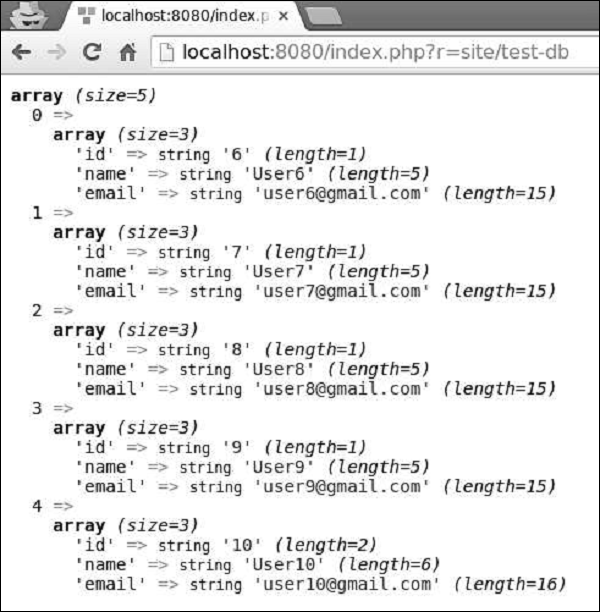The yii\db\Query class provides a set of methods for different purposes −

• all() − Returns an array of rows of name-value pairs.

• one() − Returns the first row.

• column() − Returns the first column.

• scalar() − Returns a scalar value from the first row and first column of the result.

• exists() − Returns a value indicating whether the query contains any result

• count() Returns the result of a COUNT query

• other aggregation query methods − Includes sum(\$q), average(\$q), max(\$q), min(\$q). The \$q parameter can be either a column name or a DB expression.